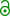## PE constraint and “politics” [R for BE/BA]

Hi GM,

» As this is a replicated study, there are only few articles for calculating the sample size.

If reference-scaling (RSABE, ABEL) is concerned, do you know any article except the two Lászlós’?1
We need simulations because the methods are implicitly sequential. Like in any framework (following a decision scheme) an analytical solution for power does not exist.
1. Estimate swR.
If <0.294* (FDA) or $$C{V_{wR}} = 100\sqrt {{e^{s_{wR}^2}} - 1} \le 30\%$$ (EMA) apply conventional, unscaled ABE.
Otherwise, continue with the respective reference-scaling method.
2. For the EMA (and others) observe an upper cap of the CVwR (i.e., don’t expand the limits beyond this point).
3. Assess the point estimate restriction.
We have two decision points for the FDA (a, c) and three (a, b, c) for the EMA…

However, the sample size estimation for ABE in replicate designs is straightforward (i.e., does not require simulations). Use sampleN.TOST() instead.

» There is no clear information about this. So that, I suppose to understand concept through R-program.

Tóthfalusi et. al.2 is a good starting point. Other references are given in the man-pages of PowerTOST.

» I thought CVswitch=0.30 for both FDA and EMA, …

Correct.

» … r_const=0.25 and 0.760 for FDA and EMA respectively …

Not quite. The switching condition θs (aka regulatory constant) is based on the regulatory standardized variation σw0. For the FDA σw0 = 0.25 and for the EMA based on CVwR 30% as $${\sigma _{w0}} = \sqrt {\log \left( {{{0.30}^2} + 1} \right)} = 0.2935604 \ldots$$
Then $${\theta _s} = \log (1.25)/\sigma _{w0} = 0.8925742 \ldots$$ (FDA) and $$0.7601283 \ldots$$ (EMA).
Note that σw0 0.25 is explicitly given by the FDA and therefore, the regulatory constant in PowerTOST is used in full precision.* On the other hand, the EMA requires the rounded 0.760 (termed k in the GL) and an upper cap for scaling at CVwR 50% (EL 69.84–143.19%). Check the conditions:

library(PowerTOST) reg_const("FDA") FDA regulatory settings - CVswitch            = 0.3 - no cap on scABEL - regulatory constant = 0.8925742 - pe constraint applied reg_const("EMA") EMA regulatory settings - CVswitch            = 0.3 - cap on scABEL if CVw(R) > 0.5 - regulatory constant = 0.76 - pe constraint applied

» … and don't know about the pe_constr.

It was introduced by all agencies following suggestions by Les Benet (see this post). It is “political” and leads to statistical troubles (essentially we are truncating the distribution and the entire concept is built on sand).
• First we have to check whether a condition is fulfilled.
FDA: Upper 95% confidence bound of $$(\overline{Y_T}-\overline{Y_R})-\theta\sigma^{2}_{wR}\leq 0$$.
EMA: 90% CI within the expanded limits $$[L,U]=\exp^{\mp k \cdot s_{wR}}$$.
• If this test passes, we have to additionally check whether the point estimate lies within 80.00–125.00%. Only then the study passes.
Note that the EMA’s method is followed in many other jurisdictions (the WHO; ASEAN States, Australia, Brazil, Egypt, the Russian Federation, the Eurasian Economic Union, the East African Community, New Zealand). Health Canada’s TGD requires an upper cap for scaling at CVwR 57.38% (EL 66.7–150.0%). Try reg_const("HC").

1. Endrényi L, Tóthfalusi L. Sample Sizes for Designing Bioequivalence Studies for Highly Variable Drugs. J Pharm Pharmaceut Sci. 2011;15(1):73–84.free resource.
2. Tóthfalusi L, Endrényi L, García-Arieta A. Evaluation of Bioequivalence for Highly Variable Drugs with Scaled Average Bioequivalence. Clin Pharmacokinet. 2009;48(11):725–43. doi:10.2165/11318040-000000000-00000

• The FDA gives σw0 0.25 and swR 0.294 (rounded!). We decided to use swR in full precision.
@Detlew: Should we change for the FDA CVswitch from 0.3 to se2CV(0.294)?

Cheers,
Helmut SchützThe quality of responses received is directly proportional to the quality of the question asked. 🚮
Science QuotesIng. Helmut Schütz